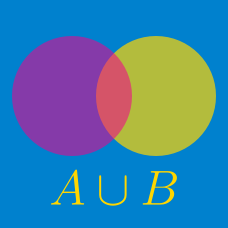Probability

# Union and Intersection

Given the sets $$A = \{2, 20, 22, 5, 13\}$$ and $$B = \{6, 9, 20, 12, 22\}$$, what is the sum of all the elements in $$A \cup B$$?

You may first choose to read the summary page Set Notation.

Given the sets $$A = \{17, 29, 65, 1, 19\}$$ and $$B = \{3, 9, 29, 101, 65\}$$, what is the sum of all the elements in $$A \cap B$$?

Details and assumptions

You may first choose to read the summary page on Set Notation.

A survey of $$80$$ students found that $$42$$ of them are wearing red and $$34$$ of them are wearing green. Furthermore, $$27$$ of them are wearing neither red nor green. What is the number of students who are wearing red, green, or red and green?

If \begin{align} A &= \{x \mid x \text{ is any positive factor of } 52\} \\ B &= \{x \mid x \text{ is any positive factor of } 338\}, \end{align}

what is $$A \cap B?$$

Given the sets $A=\{1,2,3,a,b\}, B=\{3,15,21\},$ if $$A \cup B=\{1,2,3,6,10,15,21\},$$ what is the value of $$a+b?$$

×